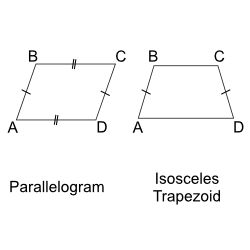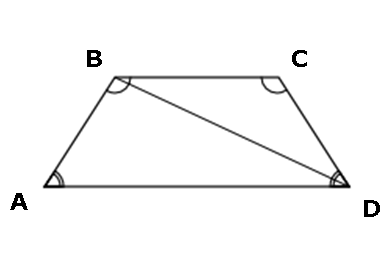We cover every section of the GMAT with in-depth lessons, 5000+ practice questions and realistic practice tests.

## Up to 90+ points GMAT score improvement guarantee

### The best guarantee you’ll find

Our Premium and Ultimate plans guarantee up to 90+ points score increase or your money back.

## Master each section of the test

### Comprehensive GMAT prep

We cover every section of the GMAT with in-depth lessons, 5000+ practice questions and realistic practice tests.

## Schedule-free studying

### Learn on the go

Study whenever and wherever you want with our iOS and Android mobile apps.

## The most effective way to study

### Personalized GMAT prep, just for you!

Correct! The issue here is whether quadrilateral \$\$ABCD\$\$ _must_ be divided into two congruent triangles by diagonal \$\$BD\$\$. By finding even one quadrilateral that cannot be divided into two congruent triangles, you have proved that both statements are insufficient. If both statements are true, then the quadrilateral is either an isosceles trapezoid (a trapezoid whose legs are of equal length) or a parallelogram.By definition, every parallelogram has congruent sides and therefore can be divided into 2 congruent triangles. However, an isosceles trapezoid meets conditions 1 and 2 but when divided by line \$\$BD\$\$, the resulting triangles are not congruent.